[Next] [Previous] [Top]

User's Guide to PHREEQC

# EQUATIONS AND NUMERICAL METHOD FOR INVERSE MODELING

In inverse modeling, one aqueous solution is assumed to react with minerals and gases to produce the observed composition of a second aqueous solution. The inverse model calculates the amounts of these gases and minerals from the difference in elemental concentrations between the two aqueous solutions. It is also possible to determine mixing fractions for two or more aqueous solutions and the mass transfers of minerals necessary to produce the composition of another aqueous solution. The basic approach in inverse modeling is to solve a set of linear equalities that account for the changes in the number of moles of each element by the dissolution or precipitation of minerals (Garrels and Mackenzie, 1967, Parkhurst and others, 1982). Previous approaches have also included an equation to conserve electrons, which forces oxidative reactions to balance reductive reactions (Plummer and Back, 1980; Parkhurst and others, 1982; Plummer and others, 1983; Plummer, 1984; Plummer and others, 1990; Plummer and others, 1991; and Plummer and others, 1994).

PHREEQC expands on these previous approaches by including a larger set of equations in the mole-balance formulation and accounts for uncertainties in the analytical data. Mole-balance equations are included for (1) each element or, for a redox-active element, each valence state of the element, (2) alkalinity, (3) electrons, which allows redox processes to be modeled, and (4) water, which allows for evaporation and dilution and accounts for water gained or lost from minerals. In addition, because alkalinity is explicitly included in the formulation, it is possible to include (5) a charge-balance equation for each aqueous solution.

The unknowns for this set of equations are (1) the mixing fraction of each aqueous solution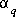, (2) the aqueous mole transfers between valence states of each redox element ar (for each redox element, the number of redox reactions is the number of valence states minus one), (3) the mole transfers of minerals and gases into or out of the aqueous solution ap, and (4) a set of unknowns that account for uncertainties in the analytical data,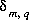. Unlike previous approaches to inverse modeling, uncertainties are assumed to be present in the analytical data, as evidenced by the charge imbalances found in all water analyses. Thus, the unknowns,, represent errors in the number of moles of each element, element valence state, or alkalinity, m, in each aqueous solution q.

The mole-balance equations (including the unknown d's) for elements and valence states are defined as follows:

(75)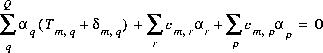,

where Q indicates the number of aqueous solutions that are included in the calculation.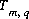is the total number of moles of element or element valence state, m, in aqueous solution q,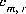is the coefficient of secondary master species m in redox reaction r,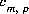is the coefficient of master species m in the dissolution reaction for phase p. The last aqueous solution, number Q, is assumed to be formed from mixing the first Q-1 aqueous solutions, and so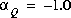. For PHREEQC, redox reactions are taken from the reactions for secondary master species in SOLUTION_SPECIES input data blocks. Dissolution reactions for the phases are derived from chemical reactions defined in PHASES and EXCHANGE_SPECIES input data blocks.

The form of the mole-balance equation for alkalinity is identical to the form of all other mole-balance equations:

(76)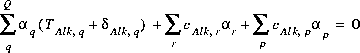,

where Alk refers to alkalinity. The difference between alkalinity and other mole-balance equations is in the meaning of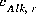and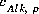. What is the contribution to the alkalinity of an aqueous solution due to aqueous redox reactions or due to the dissolution or precipitation of phases? The alkalinity contribution is defined by the sum of the alkalinities of the master species in a chemical reaction. PHREEQC definesandas follows:

(77)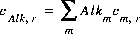,

and

(78),

where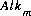is the alkalinity assigned to master species m, and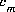is the stoichiometric coefficient of the master species m in the aqueous redox reactions and the phase dissolution reactions.

The mole-balance equation for electrons assumes that no free electrons are present in any of the aqueous solutions. Electrons may enter or leave the system through the aqueous redox reactions or through the phase dissolution reactions. However, the electron-balance equation requires that any electrons entering the system through one reaction be removed from the system by another reaction:

(79)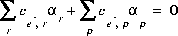where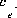represents the number of electrons released or consumed in the aqueous redox and phase dissolution reactions.

The mole-balance equation for water is

(80)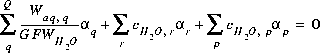,

where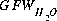is the gram formula weight for water (approximately 0.018 kg/mol),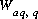is the mass of water in aqueous solution,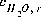is the stoichiometric coefficient of water in the aqueous redox reaction, and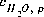is the stoichiometric coefficient of water in the dissolution reaction for phase p.

The charge-balance equations for the aqueous solutions constrain the unknown's to be such that, when the's are added to the original data, charge balance is produced in each aqueous solution. The charge balance equation for an aqueous solution is as follows:

(81)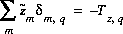,

where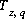is the charge imbalance in aqueous solution q calculated by a speciation calculation. The summation ranges over all elements and element valence states with non-zero concentrations and also includes a separate term for alkalinity. For alkalinity,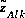is defined to be -1.0. For master species of an element or valence state, m,is defined to be the charge on the master species plus the alkalinity assigned to the master species,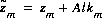. Adding the alkalinity to the charge avoids double accounting of the charge contribution of the master species. For example, the contribution of the carbonate master species to charge imbalance is zero with this definition of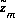; all of the contribution to charge imbalance for carbonate is included in the alkalinity term of the summation.

This formulation of the inverse problem makes sense only if the values of the's are small, meaning that the revised aqueous solution compositions (original plus's) do not deviate much from the original data. A set of inequalities insure that the values of the's are small. The absolute value of each d is constrained to be smaller than a specified value,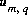:

(82)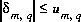.

In addition, the mixing fractions for the initial aqueous solutions (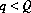) are constrained to be nonnegative,

(83)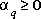,

and the final aqueous-solution mixing fraction is constrained to be -1.0 (). If phases are known only to dissolve, or only to precipitate, the mole transfer of the phases may be constrained to be nonpositive or nonnegative:

(84)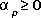,

or

(85)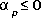.

If inorganic carbon is included in the inverse model, one additional equation is added for each aqueous solution. Unlike all other mole-balance quantities, which are assumed to vary independently, alkalinity, pH, and inorganic carbon must be assumed to co-vary. The following equation is used to relatevalues for each of these quantities:

(86)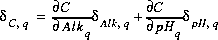,

where the partial derivatives are evaluated numerically for each aqueous solution. Inequality constraints (equation 82) are also included for carbon(+4), alkalinity, and pH for each aqueous solution.

The system of equations for inverse modeling as formulated is nonlinear because it includes the product of unknowns of the form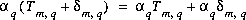. However, if the following substitution is made

(87)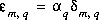,

then the mole-balance equations can be written as follows:

(88)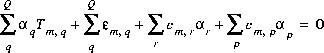,

the charge-balance equation can be rewritten as follows:

(89)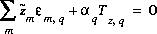,

the inequality constraints can be written as follows:

(90)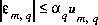,

and the relation among carbon(+4), pH, and alkalinity is

(91).

All of these equality and inequality equations are linear in the unknowns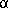and, and once the values of all of theandare known, the values ofcan be easily determined from equation 87.

This formulation of the inverse-modeling problem produces a series of linear equality and inequality constraints that need to be satisfied. The algorithm developed by Barrodale and Roberts (1980) is used to solve this optimization problem. Their algorithm performs an L1 optimization (minimize sum of absolute values) on a set of linear equations subject to equality and inequality constraints. The problem can be posed with the following matrix equations:

(92)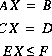.

The first matrix equation is minimized in the sense that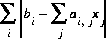is a minimum, where i is the index of rows and j is the index for columns, subject to the equality constraints of the second matrix equation and the inequality constraints of the third matrix equation. The method will find a solution that minimizes the objective functions (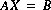) or it will determine that no feasible model for the problem exists.

Initially,is set to minimize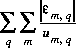. The equality constraints (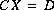) include all mole-balance, alkalinity-balance, charge-balance, electron-balance, and water-balance equations and all inorganic carbon-alkalinity-pH relations. The inequality constraints (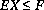) include two inequalities for each of the's, one for positive and one for negative (to account for the absolute values used in the formulation), an inequality relation for each mixing fraction for the aqueous solutions, and an inequality relation for each phase that is specified to dissolve only or precipitate only. Application of the optimization technique will determine whether any inverse models exist that are consistent with the constraints.

Thus, we may be able to find one set of mixing fractions and phase mole transfers (plus associated's) that satisfy the constraints. Ignoring the values of the's and redox mole transfers (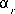), let the set of nonzeroand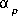(mixing fractions and phase mole transfers) uniquely identify an inverse model. The magnitude of the's is not considered in the identity of an inverse model, only the fact that a certain set of the's are nonzero. (At this point, little significance should be placed on the exact numbers that are found, only that it is possible to account for the observations using the stated aqueous solutions and phases.) But could other sets of aqueous solutions and phases also produce feasible inverse models? An additional algorithm is used to find all of the unique inverse models.

Assuming P phases and Q aqueous solutions, we proceed as follows: If no feasible model is found when all Q aqueous solutions and P phases are included in the equations, we are done and no feasible models exist. If a feasible model is found, then each of the phases in this model is sequentially removed and the remaining set of aqueous solutions and phases is tested to see if a feasible model can be found. If a feasible model is not found when excluding a particular phase, then it is retained in the model, else it is discarded. After each phase has been tested, the phases that remain constitute a "minimal" model, that is, none of the phases can be removed and still obtain a feasible model. Three lists are kept during this process, each feasible model is kept in one list, each infeasible model is kept in another list, and each minimal model is kept in a third list.

Next, each combination of P-1 phases is tested for feasible models as follows: If the set of aqueous solutions and phases is a subset of an infeasible model or a subset of a minimal model, the model is skipped. If only minimal models are to be found (-minimal in INVERSE_MODELING keyword data block), the model is also skipped if it is a superset of a minimal model. Otherwise, the inverse problem is formulated and solved using the set of aqueous solutions and the P-1 phases in the same way as described above, maintaining the three lists during the process. Once all sets of P-1 phases have been tested, the process continues with sets of P-2 phases, and so on until the set containing no phases is tested or until, for the given number of phases, every set of phases tested is either a subset of an infeasible model or a subset of a minimal model.

At this point, the entire process is repeated using each possible combination of one or more of the Q aqueous solutions. Although the process at first appears extremely computer intensive, most sets of phases are eliminated by the subset and superset comparisons, which are very fast. The number of models that are formulated and solved by the optimization methods are relatively few. Also the process has the useful feature that if no feasible models exist, this is determined immediately with the first invocation of the optimization procedure. During all of the testing, whenever a feasible model is found, it is printed to the output device or optionally, only the minimal models are printed to the output device.

An alternative formulation of the objective functions can be used to determine the range of mole transfer for each aqueous solution and each phase that is consistent with the specified uncertainties. For the "range" calculation (-range in INVERSE_MODELING keyword data block), the equations for a given model are solved twice for each aqueous solution and phase in the model, once to determine the maximum value of the mixing fraction or mole transfer and once to determine the minimum value of the mixing fraction or mole transfer. In these calculations, the's are not minimized, instead, the single objective function for maximization is simply

(93)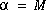,

and in the minimization case,

(94)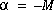,

whererefers to eitheror. By default, the value of M is 1000. The optimization method will try to minimize the difference betweenand 1000 and -1000. The number 1000 should be large enough for most calculations, but it is possible that the method will fail, causingto be equal to 1000 instead of a true maximum, in some evaporation problems, where a mixing fraction of greater than 1000 is conceivable. The value of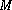may be changed with a parameter in the -range identifier.

For data input to PHREEQC, identifiers in the INVERSE_MODELING keyword data block are used for the selection of aqueous solutions (-solutions), uncertainties (-uncertainties and -balances), reactants (-phases), mole-balance equations (-balances), range calculations (-range) and minimal models (-minimal). aqueous solution compositions are defined with the SOLUTION keyword data block and reactants must be defined with PHASES or EXCHANGE_SPECIES keyword data blocks. (See Description of Data Input.)

User's Guide to PHREEQC - 07 MAY 96
[Next] [Previous] [Top]